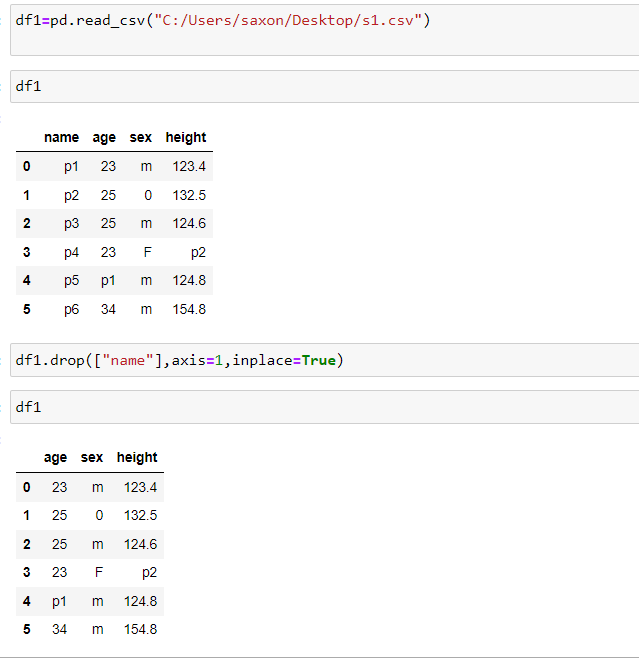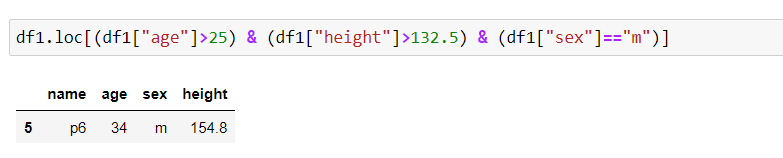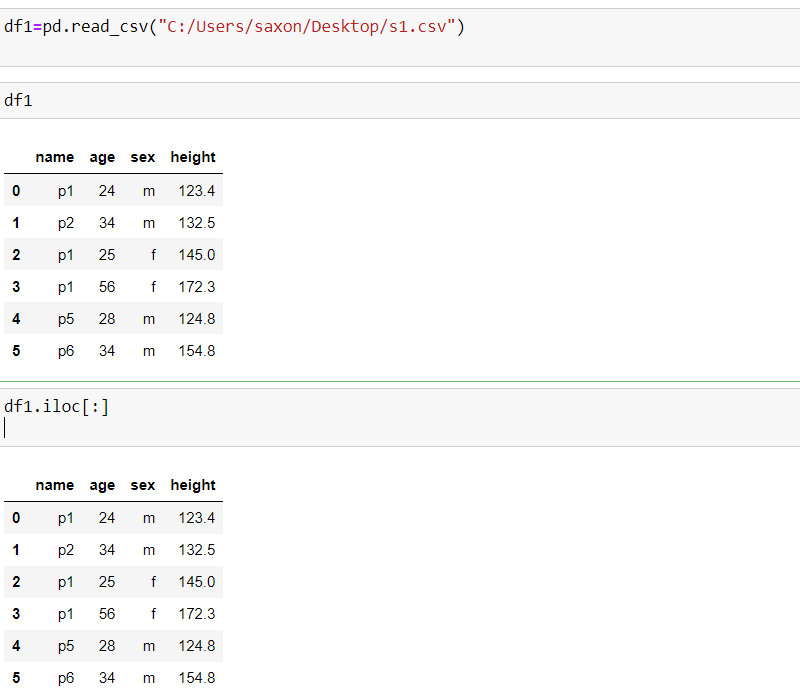top of page
Search

# Pandas Part 5

## How To Access Columns From A Data Frame

• We can access a column from a dataframe by using [] brackets

• We can use single [] for accessing a single column or use [[]] for accessing single or multiple columns

Example 1## How To Delete A Column From A Data Frame

• We can use drop() function or can use pop() or we can use del keyword also

### drop()

• drop() function can be used to remove rows as well as columns

• So if i want to remove column by using drop() we have to use axis parameter as 1 (1 for rows 0 for columns)

Example 2• When trying to make changes to a Pandas dataframe using a function,like drop() here we use 'inplace=True' if we want to commit the changes to the orginal dataframe or else default will be inplace=False no changes to orginal dataframe

Example 3### pop()

• By using pop() also we can remove a column

• This functions directly affects the original data

Example 4### del keyword

• We can use delete keyword to delete a column

Example 5• del can also be used to delete the entire dataframe

Example 6## How To Add A Column To An Existing Data Frame

• By using simple accessing method we can add a column to a dataframe

• First it will check whether that particular column label is there or not if it matches with any column label in the dataframe then it will replace the existing column values else creates a new column with the given values

Example 7Example 8• if the appended column length does not matches with the dataframe column length then it will try to extend the appended column length with nan values to match with the dataframe length

Example 9## How To Select Rows From A Data Frame

• iloc and loc indexing are two main technique to select rows from a dataframe

• iloc -- integer based

• loc -- label based

#### loc

• loc is label-based, here we use labels (names) of columns and rows to filter it out

• example, let’s say we are search for the rows whose index is 1, 5 or 15. Here we will get only the rows who's index are 1, 5 and 15 not 1 row 5th row or 15 row

• By using loc we can filter the data by some condition

Example 10Example 11

Using loc, we can also slice the pandas dataframe . In loc when we do slicing the end index is also included### Find all the rows based on any condition in a column

• loc can also be used to filter out the rows based on the condition applied to columns

• we can solve types of queries with a simple line of code using DataFrame.loc[]. We just need to pass the condition within the loc statement.

Example 12**In the 1 ex above we filtered out all the rows in our dataset where age is greater than 25

**In the 2 ex above we filtered out all the rows in our dataset where sex is equal to m

### Find all the rows with more than one condition

• We can also use multiple conditions to filter our data

• For example finding out all the rows in our dataset where age is greater than 25 and sex equal to m

Example13Example 14Example 15

** When we are applying two or more conditions pass each condition in this format

(condition1) & (condition2)

** other wise we will get and error trying to pass multiple conditons** Use & and | symbols for "and" and for "or " condition

** we will get an error if use "and" or "for" keyword between each condition

Example 16### Select only the required columns with a condition

• We can also select the columns that are required of the rows that satisfy our condition

• For example, if our dataset contains many columns and we want to view only a few of them, then we can add a list of columns after the condition within the loc statement itself

Example 17** pass it as a list

Example 18### Update the values of a particular column on selected rows

• Some time we have to update some values in our dataset based on a certain condition. So here we we just need to specify the condition followed by the target column and then assign the value with which we want to update

Example 19Example 20

Here we updated the sex column values with "f" where age > 25

Here we updated the sex and age column values with "f" and 50 where age > 25#### iloc

• iloc is integer-based, here we we have to specify rows and columns by their integer index.

• example, let’s say we are searching for the rows whose index is 1, 5 or 15. Here we will get the 1 ,5 and 15 rows not he rows with label 1,5 and 15

Example 21Example 22

Using iloc, we can also slice the pandas dataframe . In iloc when we do slicing the end index is not includedExample 23

With iloc we cant filter out the data### Select rows with indices using iloc

• When we are using iloc, we need to specify the rows and columns by their integer index

Example 24### Select rows with particular indices and particular columns

• if we wanted to select a few columns from the dataset We can also do this by using the iloc function. When we use iloc we need to provide the index number of the column instead of the column name

Example 25Example 26

• when we use iloc always we need to provide the index number of the column instead of the column name or else we get index error